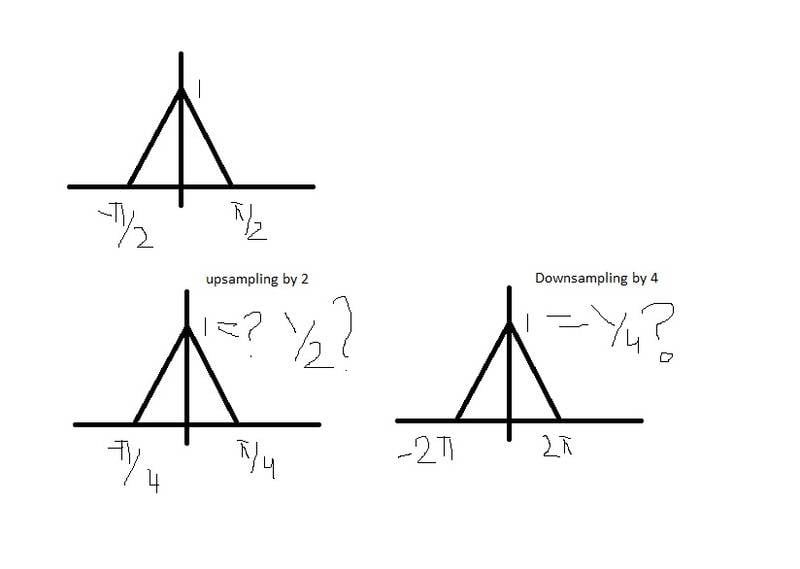# Signal Processing: Up and Down Sampling

coolrp

## Homework Statement

Does the upsampling and downsampling have any effect on the amplitude?

consider the following X(e^jw)## Homework Equations

what will be the amplitude of the signals? do they remain 1 or are they to be divided/multiplied by L/M, L being upsampling factor and M being downsampling factor?

Homework Helper
Gold Member
What is "up" & "down" sampling? Do you mean oversampling and undersampling? With relation to Nyquist rate?

coolrp
up and down sampling means increasing or decreasing the sampling frequency!

Homework Helper
Gold Member
ƩIncreasing or decreasing the sampling frequency for a given input signal x(t) does not change the amplitude opf the samples.

x(t) after sampling is Ʃx(nT)δ(t - nT) so x(nT) is the only thing that determines amplitude.